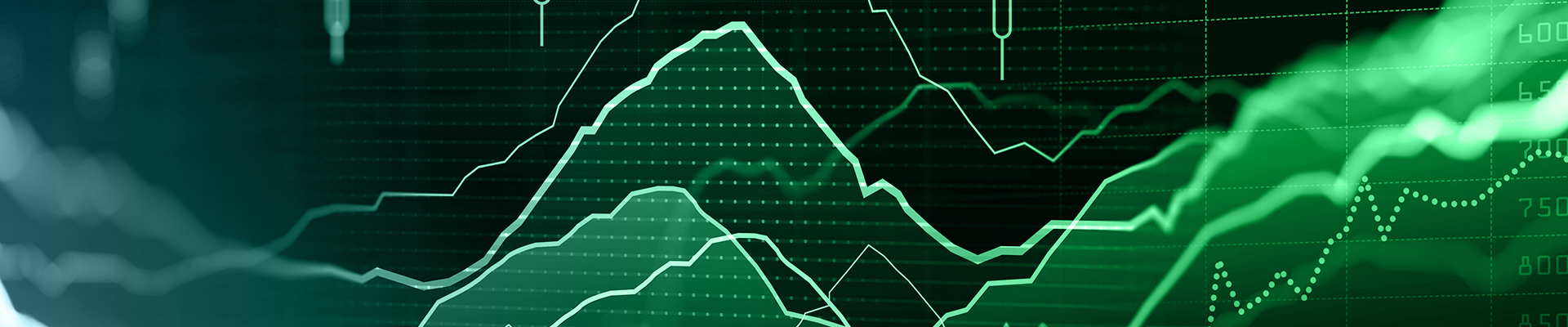# How To Trade With Stochastic?

## Introduction

Technical Indicators are mathematical formulas calculated from price data to provide traders with some information that cannot be easily shown from the pure prices like momentum and volatility. Indicators have two major classifications trend-following, like Moving averages and Bollinger Bands, and Oscillators like RSI and Stochastics.

## What is a Stochastic?

A stochastic oscillator is a momentum indicator comparing the current closing price of a security to a range of its prices over a certain period. This is invented by George C. Lane. Stochastic has two types Fast stochastics and slow stochastics.

It oscillates around level 50 between 0 and 100. As above 20 called an overbought area And below 20 is called an oversold area. It consists of two lines, K% and D%, as shown in Figure (1)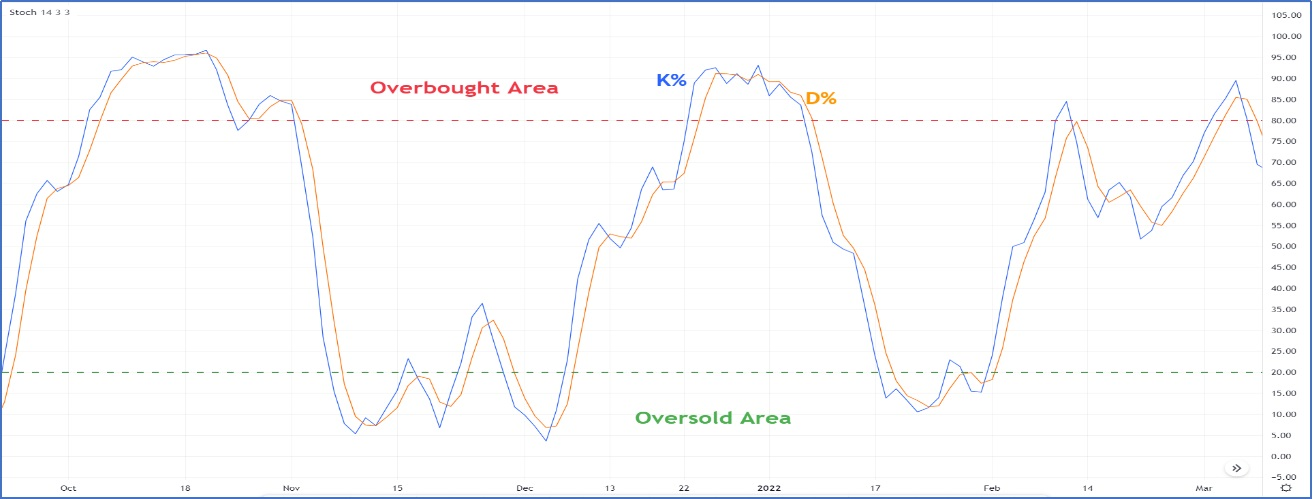Figure 1

×## Types of Stochastic

1-Fast Stochastics.

%K = (Current Close – Lowest of Range) / (Highest of Range – Lowest of Range) Range is how many time periods that stochastics is calculated over

D% = SMA (3) of %K

2-Slow Stochastics

%K = D% (of the Fast Stochastics)

D% = SMA (3) of %K

Stochastic gives a buy signal when there is a positive crossover between the k% line and D% line and sell signals when there is a negative crossover between the k% line and D% line.

You cannot use the Stochastic signal alone without considering trend analysis because every market phase has a different trading characteristic. In an uptrend, using Stochastic to time the buy signal will always be the best Stochastic trading strategy, and in a downtrend, using Stochastic to time the short signal.

### How to trade using the Stochastic indicator in an uptrend?

During an uptrend, buyers always have control over price movement, and the best trades are always around the end of the downward retracement, where the best risk-reward ratio is present.

In a strong uptrend Stochastic indicator may not reach the oversold area, so when Stochastic has a positive crossover in the zone, 40:20 could be a buying signal, as shown in figure (2)

1-In a Normal uptrend Stochastic indicator may reach an oversold area below 20, so the crossover could be a buying signal, as shown in figure (3)

When Stochastic moves above level 80, what we call an overbought area in the uptrend does not mean it is an excellent time to sell it means buyers are aggressive, and that might lead to some relief in the price movement due to some profit-taking. So, when Stochastic bounced down from overbought, it is an excellent time to take profits for long positions, not to initiate a new short position.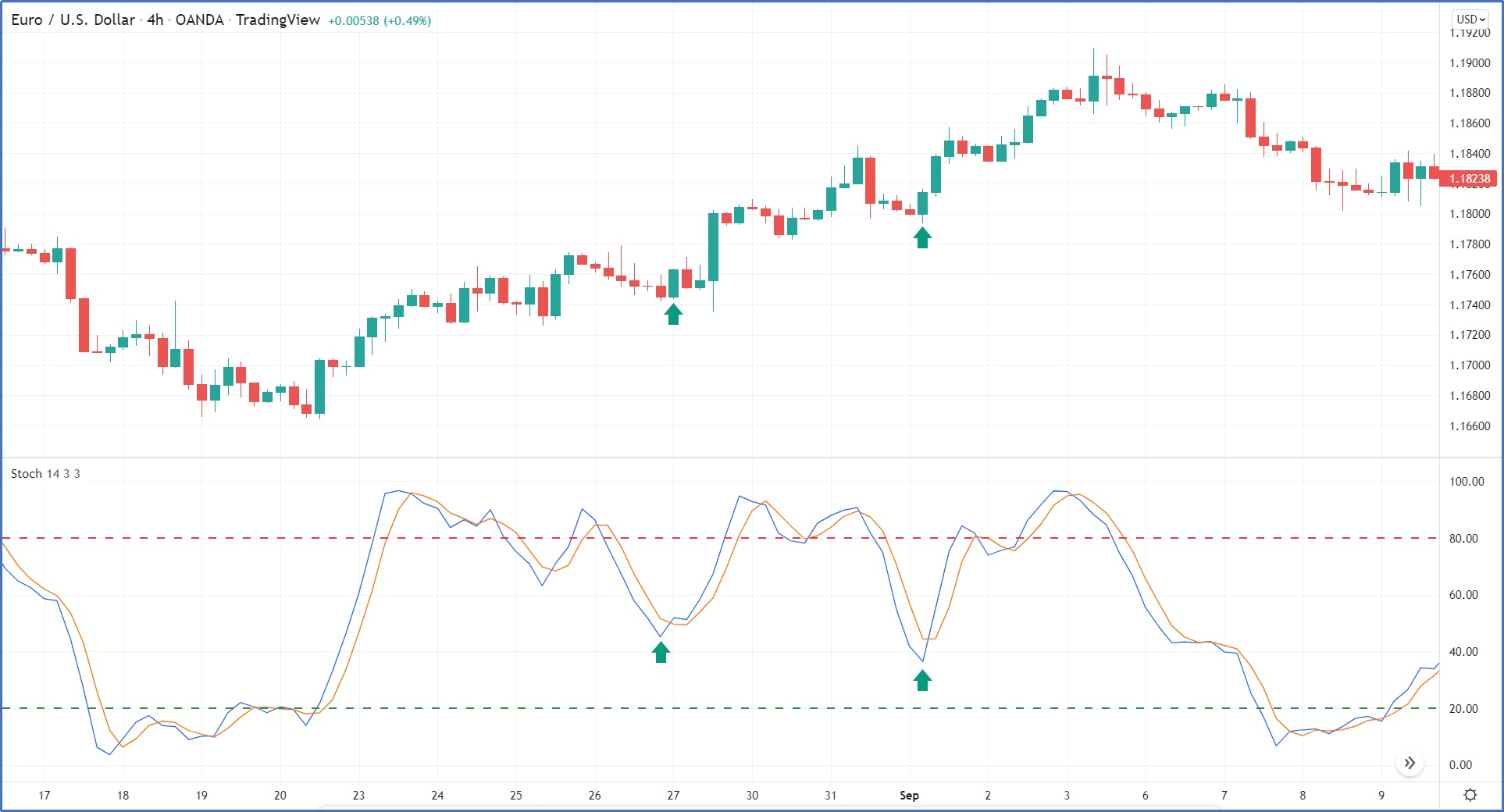Figure 2

×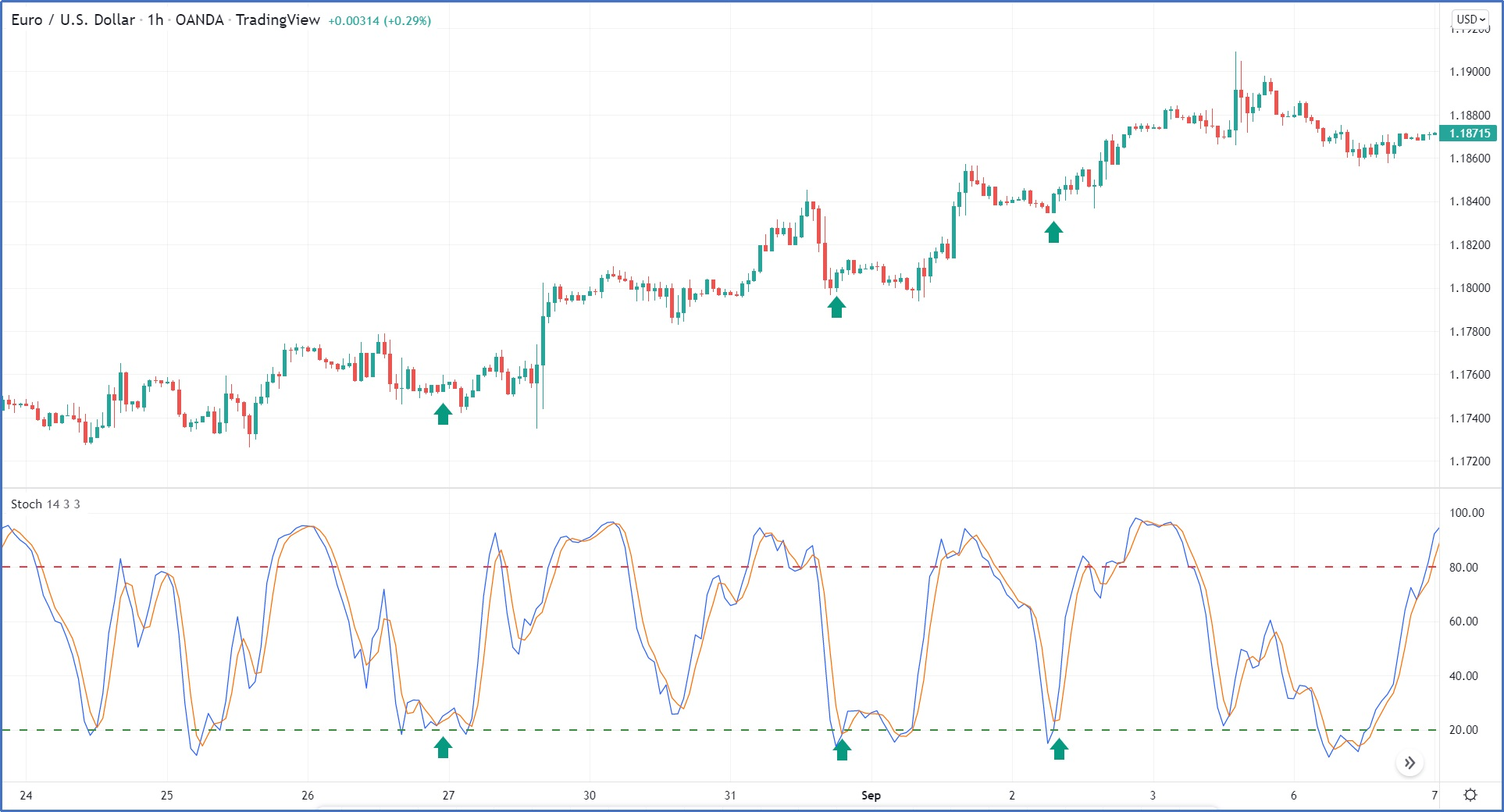Figure 3

×### How to trade using Stochastic indicator in Downtrend?

During a downtrend, sellers always have control over price movement, and the best trades are always around the end of the upward retracement, where the best risk-reward ratio is present.

1-In a strong downtrend Stochastic indicator may not reach the overbought area, so when Stochastic has a negative crossover in the zone, 60:70 could be a selling signal, as shown in figure (4)

2-In a Normal downtrend Stochastic indicator may reach an overbought area above 80, so the crossover could be a sell signal, as shown in figure (5)

When Stochastic moves below level 20, what we call an oversold area in the downtrend does not mean it is a good time to buy. It means sellers are aggressive, which might lead to some relief in the price movement due to some profit-taking. So, when Stochastic bounced up from oversold, it is a good time to take profits for short positions, not to initiate a long position.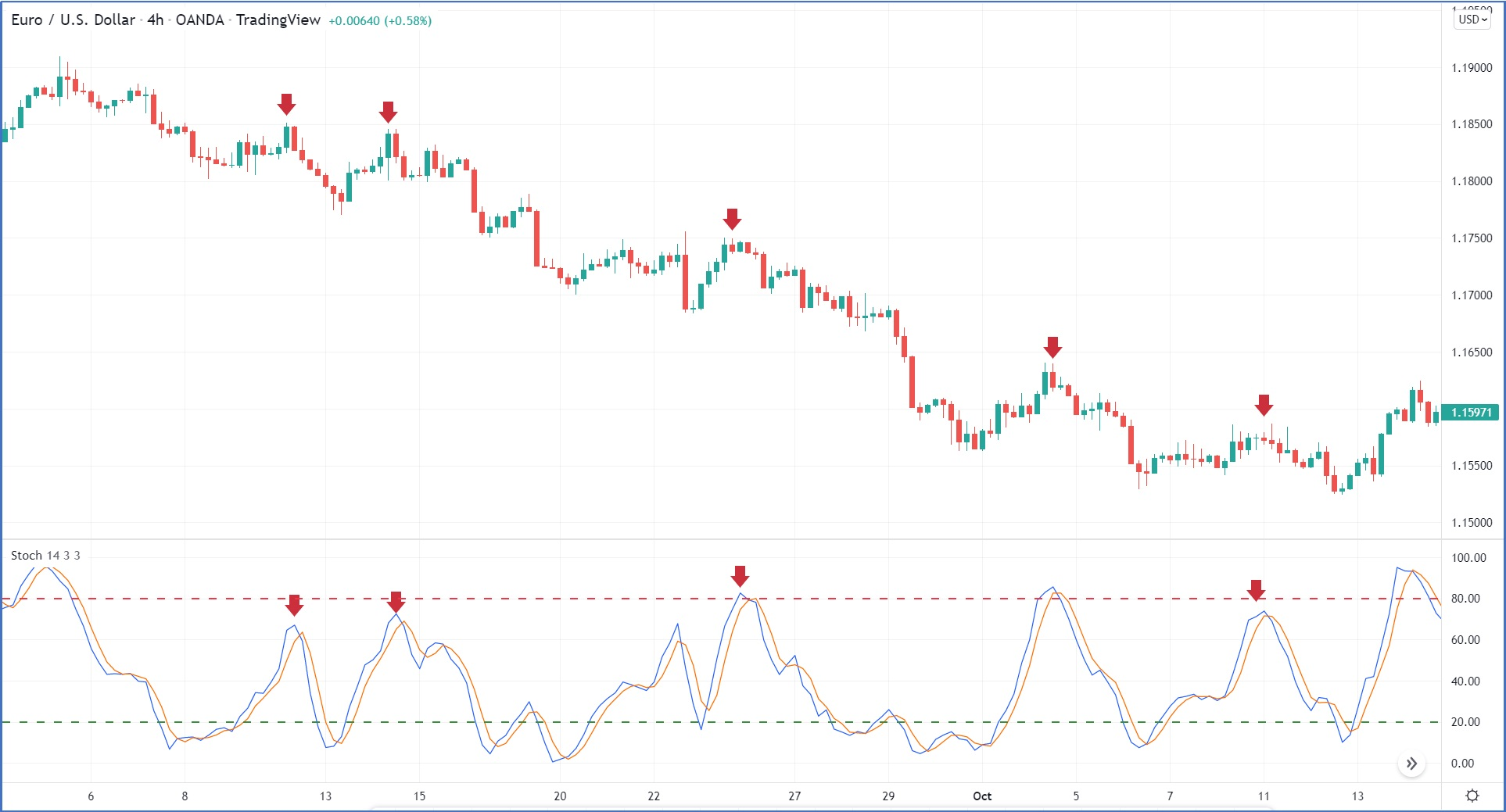Figure 4

×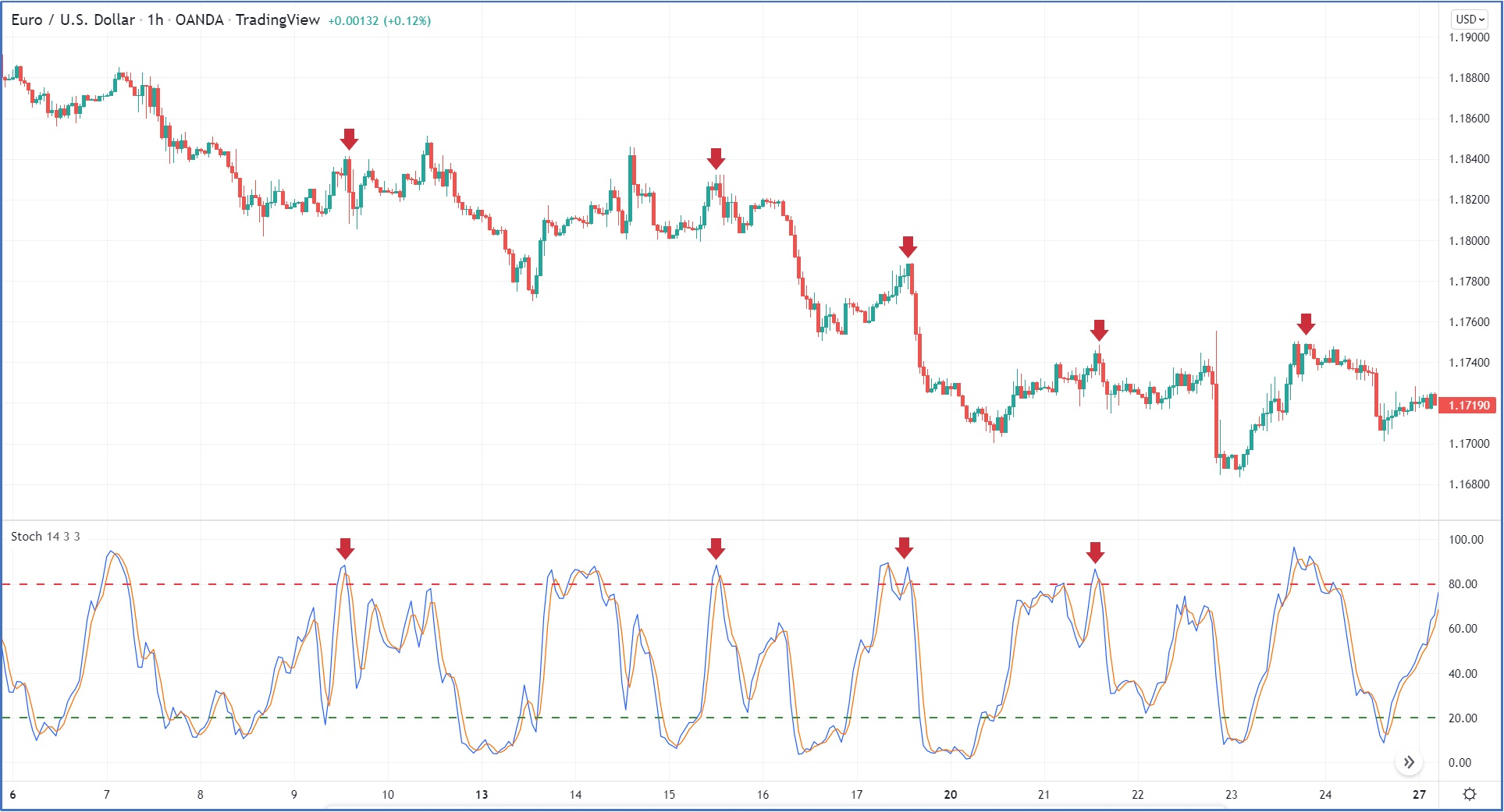Figure 5

×### How to trade using a Stochastic indicator in Sideways Movements?

Sideways movement occurs when there is an equilibrium between buyers and sellers, so when RSI bounce down around the overbought area could be a sell signal, and when RSI bounced up around the oversold area, it could be used as a buy signal, as shown in figure (6)Figure 6

×## What is a divergence using Stochastic?

Most of the time, the Stochastic indicator follows the price movement, but when it does not, we call this a Divergence which indicates a weakness in the current trend.

There are two types of divergence:

### Positive Divergence

It occurs when price make a lower low, but the indicator makes a higher low, it means the current seller exhausted and loses momentum, and a correction might occur, as shown in figure (7)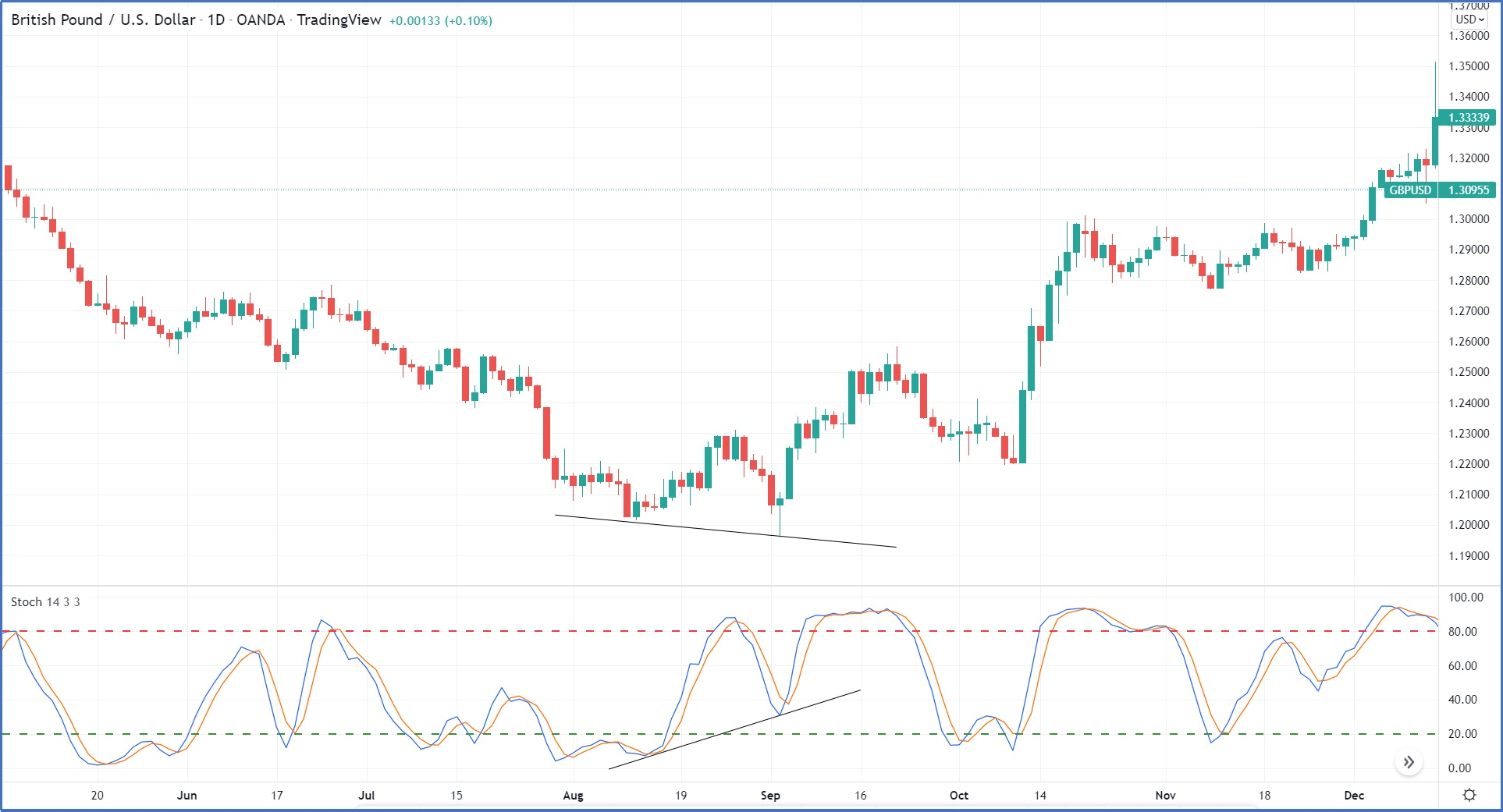Figure 7

×### Negative Divergence

It occurs when price make a higher high, but the indicator makes a lower high, which means the current buyer is exhausted and loses momentum, and correction might occur, as shown in figure (8).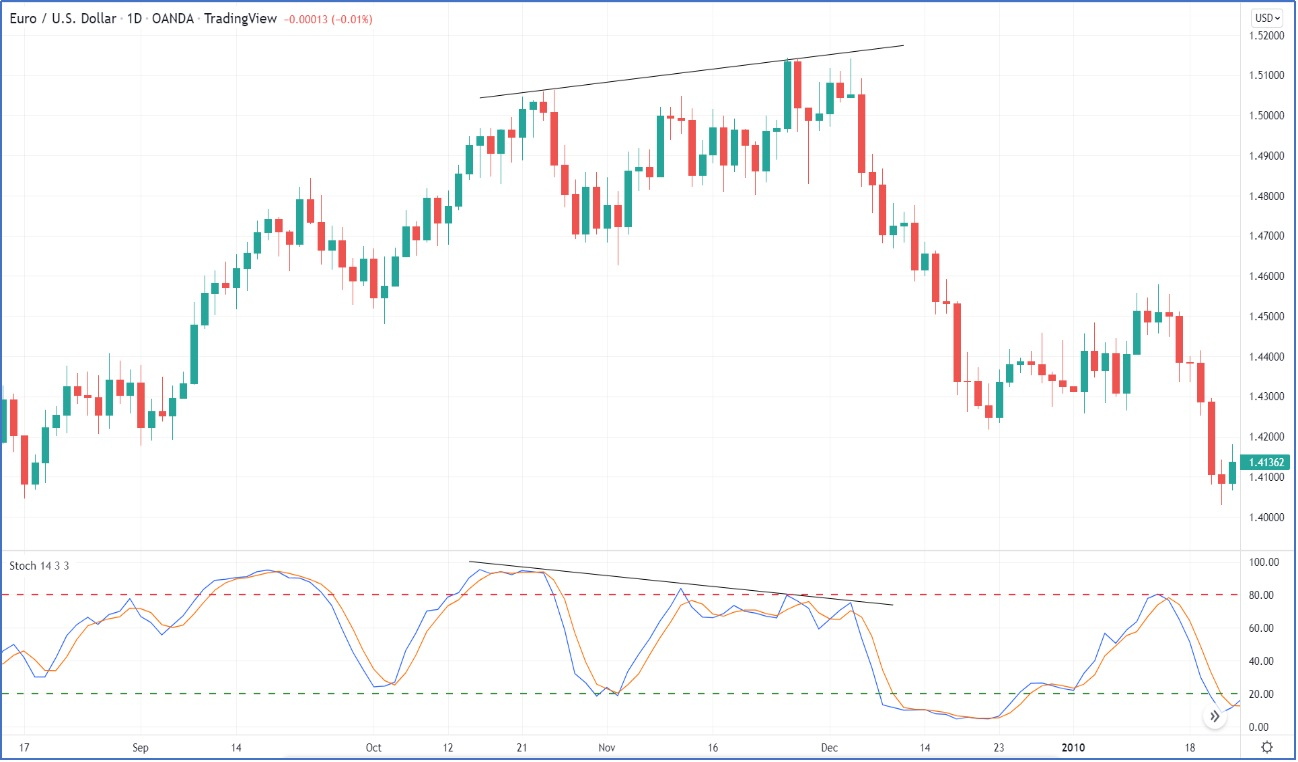Figure 8

×A divergence signal is a mean reversion because the trader takes a position opposite to the current trend. For example, when a negative divergence appears in an uptrend short position could be taken, and when a positive divergence appear long position could be taken because divergence is an indication of a short-term correction. Divergence signals have a 1:1 risk-reward ratio but are highly likely.

When Stochastic shows a positive divergence, wait for any candlestick reversal pattern as a buy signal with stop loss below the candlestick pattern and using BB moving average or 50% retracement level as a target, as shown in figure(10)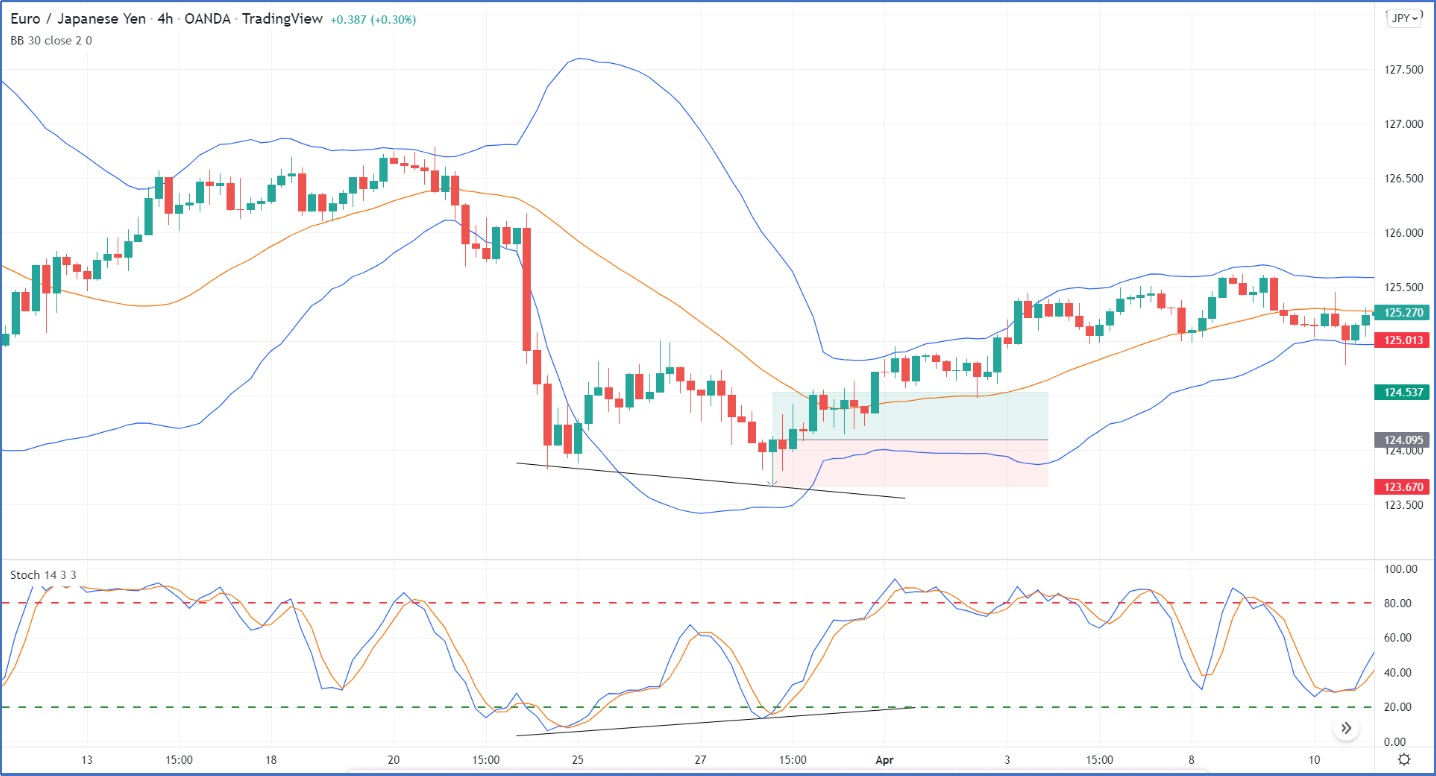Figure 10

×When Stochastic shows a negative divergence, wait for any candlestick reversal pattern as a sell signal with stop loss above the candlestick pattern and using BB moving average or 50% retracement level as a target, as shown in figure (11)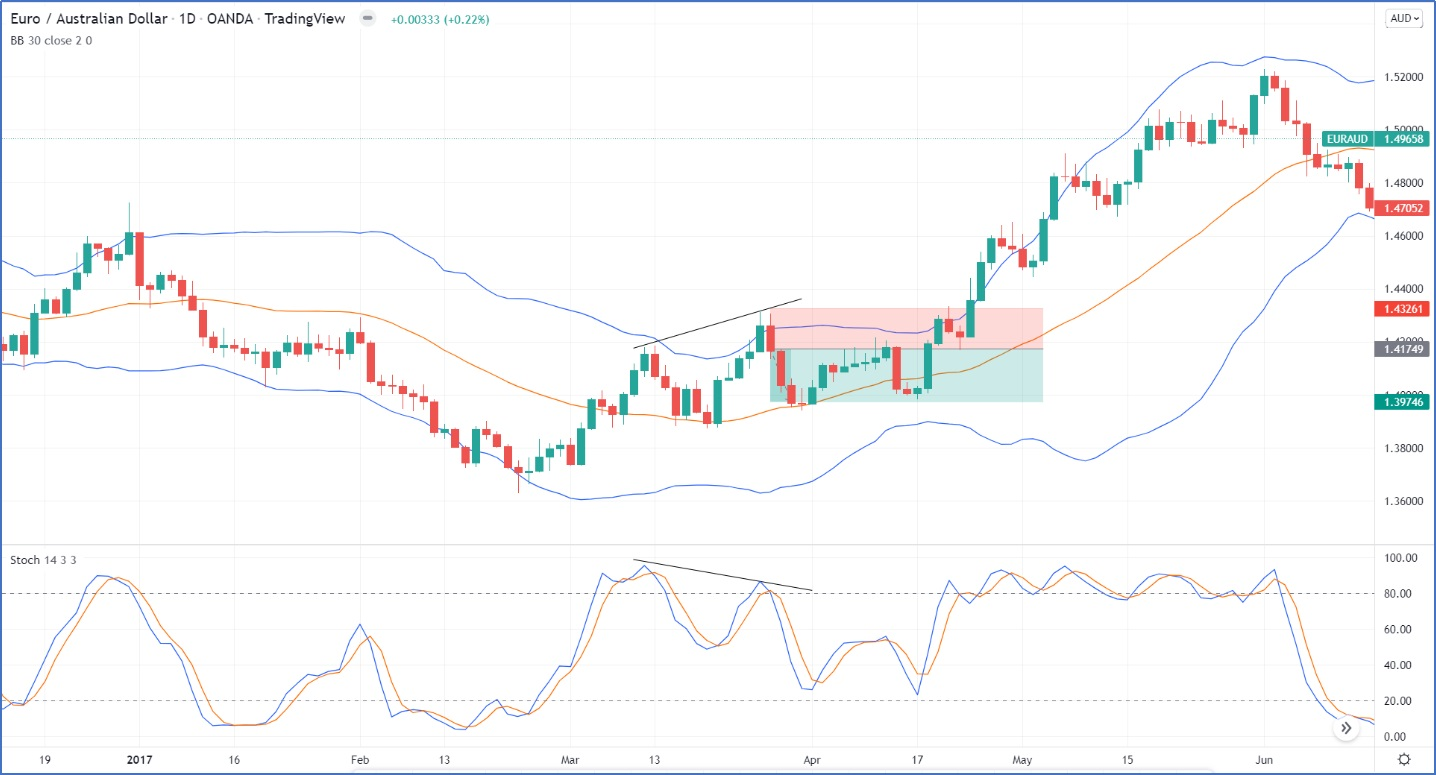Figure 11

×## How to combine Stochastic and MACD indicator?

We can combine stochastic and MACD to improve the quality of trading signals by using stochastic for entries and MACD as signals filters.

1. When Stochastic moves above level 80, that indicates a strong uptrend
2. When stochastics bounces down below level 80, that indicates a downward correction is beginning.
3. When stochastics has a positive crossover around level 50, that means that correction is over
4. MACD will be used as a trending indicator, so buying signals will be executed only when the MACD line moves above the signal line

As shown in the figure (12), an example of using Stochastic with MACD to improve the quality of buy signal.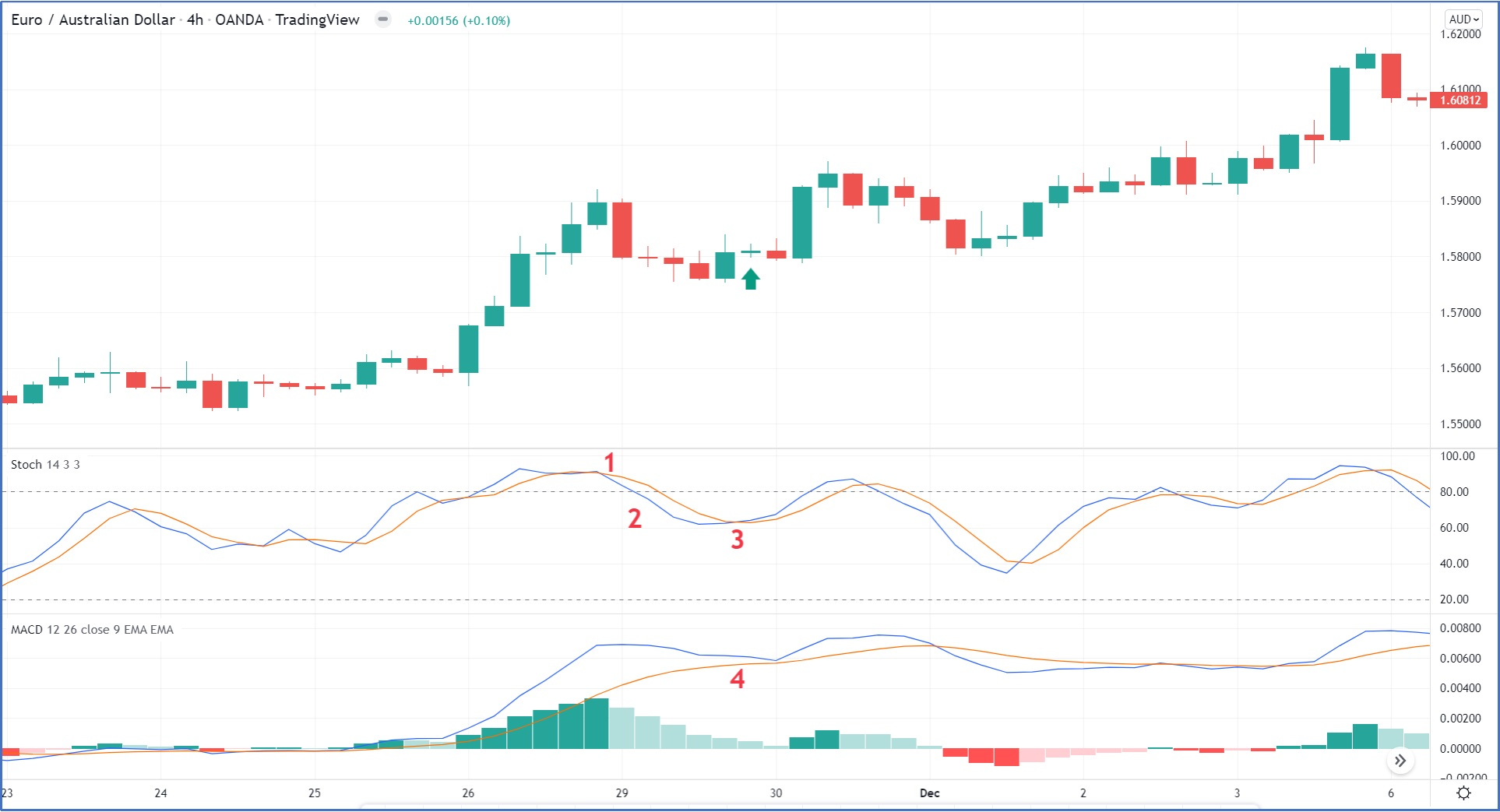Figure 12

×